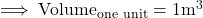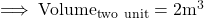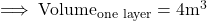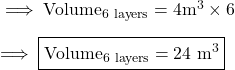## What is the volume of the prism? A rectangular prism with cubic units arranged in two rows of two with six total layers. Each cu

Question

What is the volume of the prism?

A rectangular prism with cubic units arranged in two rows of two with six total layers. Each cubic unit is one cubic meter.

14 m3
24 m3
30 m3
44 m3

in progress 0
7 months 2021-07-23T14:12:59+00:00 1 Answers 14 views 0

24 m³

Step-by-step explanation:

We need to find the volume of the prism. Given that , rectangular prism with cubic units arranged in two rows of two with six total layers . And the volume of each unit is 1 .

Volume of one unit :By Unitary Method :

Volume of two cubes , will be :-Volume of one layer :Volume of six layers :